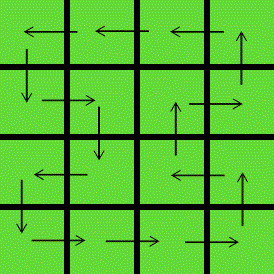Competitions

PP1 and a bit more

Ada's classroom contains n * m tables distributed in a grid with n rows and m columns. Each table is occupied by exactly one student.

Before starting the class, the teacher decided to shuffle the students a bit. After the shuffling, each table should be occupied by exactly one student again. In addition, each student should occupy a table that is adjacent to that student's original table, i.e. immediately to the left, right, top or bottom of that table.

Is it possible for the students to shuffle while satisfying all conditions of the teacher?

Input

The first line contains the number of test cases t. Each of the next t lines contains two integers n and m (2n, m1000).

Output

For each test case, print a single line containing the string "YES" if it is possible to satisfy the conditions of the teacher or "NO" otherwise.Time limit 1 second
Memory limit 128 MiB
Input example #1
2
5 5
4 4
Output example #1
NO
YES